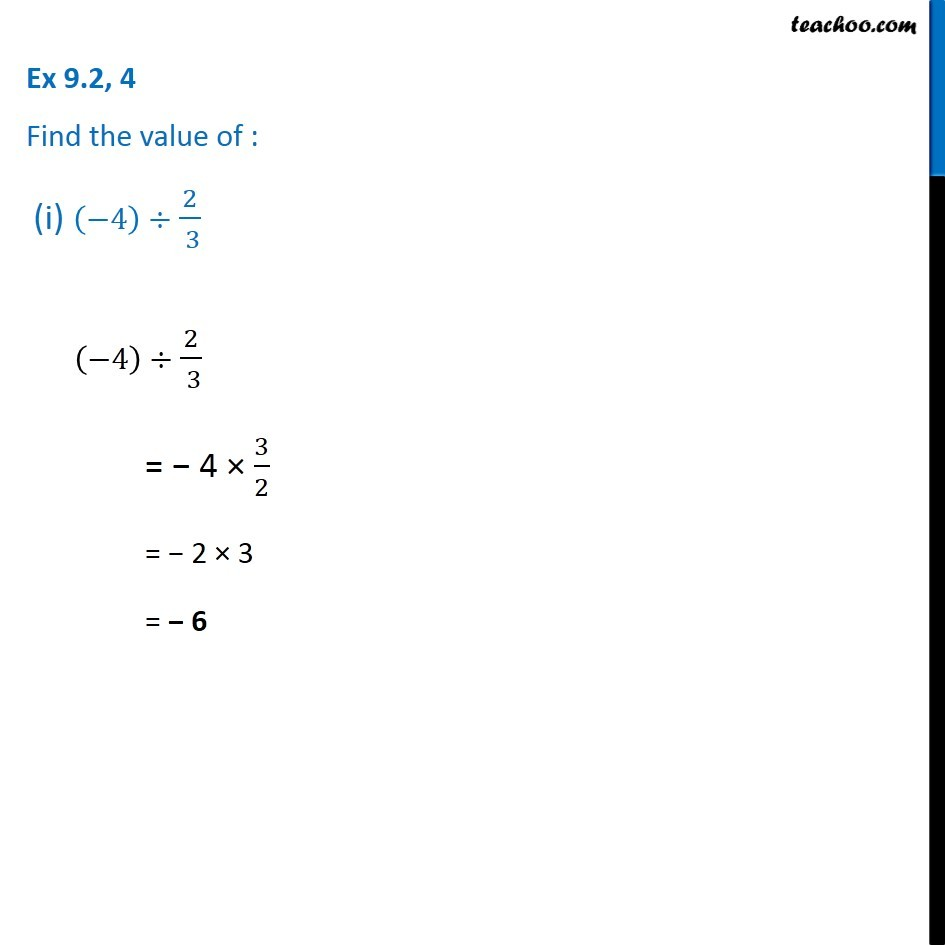1. Chapter 9 Class 7 Rational Numbers
2. Concept wise
3. Division of rational numbers

Transcript

Ex 9.2, 4 Find the value of : (i) (−4)÷ 2/( 3) (−4)÷ 2/( 3) = − 4 × 3/2 = − 2 × 3 = − 6